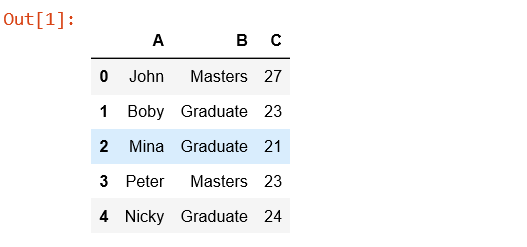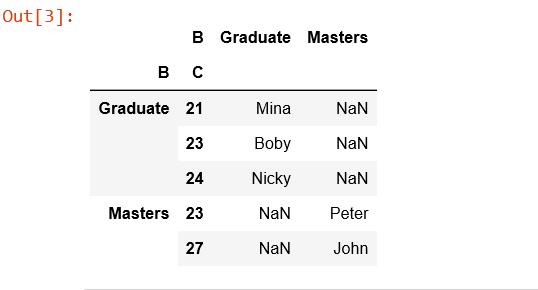# Python | Pandas.pivot_table()

pandas.pivot_table(data, values=None, index=None, columns=None, aggfunc=’mean’, fill_value=None, margins=False, dropna=True, margins_name=’All’) create a spreadsheet-style pivot table as a DataFrame.

Levels in the pivot table will be stored in MultiIndex objects (hierarchical indexes) on the index and columns of the result DataFrame.

`Parameters:`

data : DataFrame
values : column to aggregate, optional
index: column, Grouper, array, or list of the previous
columns: column, Grouper, array, or list of the previous

aggfunc: function, list of functions, dict, default numpy.mean
-> If list of functions passed, the resulting pivot table will have hierarchical columns whose top level are the function names.
-> If dict is passed, the key is column to aggregate and value is function or list of functions

fill_value[scalar, default None] : Value to replace missing values with
margins[boolean, default False] : Add all row / columns (e.g. for subtotal / grand totals)
dropna[boolean, default True] : Do not include columns whose entries are all NaN
margins_name[string, default ‘All’] : Name of the row / column that will contain the totals when margins is True.

`Returns:` DataFrame

Code:

 `# Create a simple dataframe ` `  `  `# importing pandas as pd ` `import` `pandas as pd ` `import` `numpy as np ` `  `  `# creating a dataframe ` `df ``=` `pd.DataFrame({``'A'``: [``'John'``, ``'Boby'``, ``'Mina'``, ``'Peter'``, ``'Nicky'``], ` `      ``'B'``: [``'Masters'``, ``'Graduate'``, ``'Graduate'``, ``'Masters'``, ``'Graduate'``], ` `      ``'C'``: [``27``, ``23``, ``21``, ``23``, ``24``]}) ` `  `  `df ``# Simplest pivot table must have a dataframe ` `# and an index/list of index. ` `table ``=` `pd.pivot_table(df, index ``=``[``'A'``, ``'B'``]) ` ` `  `table ``# Creates a pivot table dataframe ` `table ``=` `pd.pivot_table(df, values ``=``'A'``, index ``=``[``'B'``, ``'C'``], ` `                         ``columns ``=``[``'B'``], aggfunc ``=` `np.``sum``) ` ` `  `table `My Personal Notes arrow_drop_upCheck out this Author's contributed articles.

If you like GeeksforGeeks and would like to contribute, you can also write an article using contribute.geeksforgeeks.org or mail your article to contribute@geeksforgeeks.org. See your article appearing on the GeeksforGeeks main page and help other Geeks.

Please Improve this article if you find anything incorrect by clicking on the "Improve Article" button below.

Article Tags :

1

Please write to us at contribute@geeksforgeeks.org to report any issue with the above content.